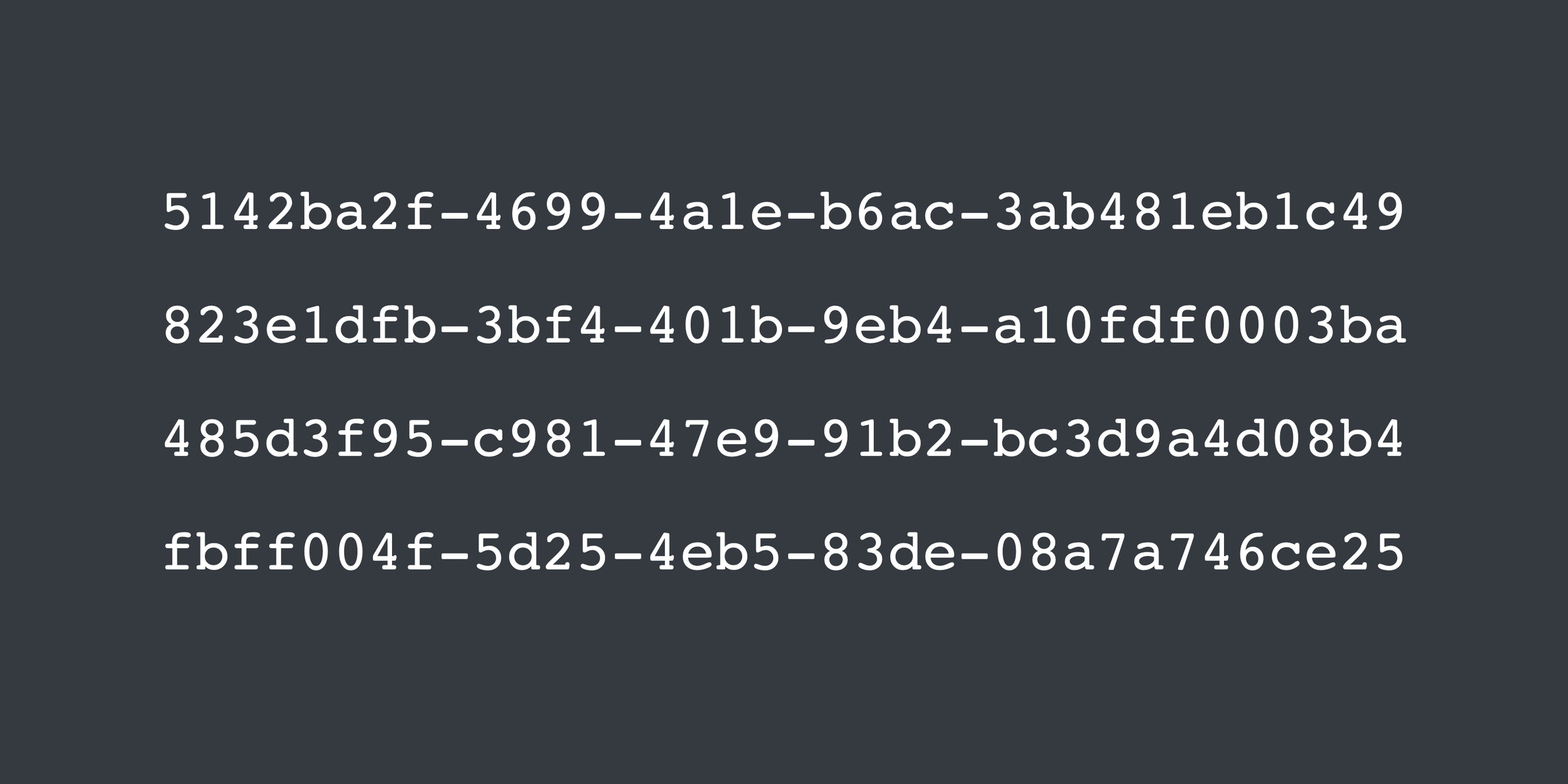# Generating v4 UUIDs in MySQLChristian Emmer
Nov 13, 2020 · 3 min readMySQL's `UUID()` function generates v1 UUIDs , which have a time component that make them unevenly distributed over short periods of time. We can define our own function to generate v4 UUIDs , which are random and therefore more evenly distributed.

Check out "Why You Should Use UUIDs for Your Primary Keys" for a reason to use UUIDs in databases.

Why You Should Use UUIDs for Your Primary Keys

Jan 30, 2020 · 4 min read

Universally unique identifiers (UUIDs) are 128-bit (16-byte) numbers that are designed to be globally unique, and as a result they make for great primary keys.## A custom `uuid_v4()` function

Here's the function, with comments explaining each group:

``````CREATE FUNCTION uuid_v4() RETURNS CHAR(36)
BEGIN
-- 1st group is 8 characters = 4 bytes
SET @g1 = HEX(RANDOM_BYTES(4));

-- 2nd group is 4 characters = 2 bytes
SET @g2 = HEX(RANDOM_BYTES(2));

-- 3rd group is 4 characters = 2 bytes, starting with a: 4
SET @g3 = CONCAT('4', SUBSTR(HEX(RANDOM_BYTES(2)), 2, 3));

-- 4th group is 4 characters = 2 bytes, starting with a: 8, 9, A, or B
SET @g4 = CONCAT(HEX(FLOOR(ASCII(RANDOM_BYTES(1)) / 64) + 8), SUBSTR(HEX(RANDOM_BYTES(2)), 2, 3));

-- 1st group is 12 characters = 6 bytes
SET @g5 = HEX(RANDOM_BYTES(6));

RETURN LOWER(CONCAT(@g1, '-', @g2, '-', @g3, '-', @g4, '-', @g5));
END;``````

Here's a version without variables, to remove any overhead they might add:

``````CREATE FUNCTION uuid_v4() RETURNS CHAR(36)
BEGIN
RETURN LOWER(CONCAT(
HEX(RANDOM_BYTES(4)),
'-', HEX(RANDOM_BYTES(2)),
'-4', SUBSTR(HEX(RANDOM_BYTES(2)), 2, 3),
'-', HEX(FLOOR(ASCII(RANDOM_BYTES(1)) / 64) + 8), SUBSTR(HEX(RANDOM_BYTES(2)), 2, 3),
'-', hex(RANDOM_BYTES(6))
));
END;``````

This uses `RANDOM_BYTES()` instead of `RAND()` because the former is non-deterministic and therefore more cryptographically secure, resulting in fewer UUID collisions in the end.

`RANDOM_BYTES()` was introduced in MySQL v5.6.17 (2014) , and is currently not available in MariaDB.

## Putting it all together

Here's an example where a table `users` uses UUIDs for its primary key. Using `CHAR(36)` simplifies this example, but see "Making UUIDs More Performant in MySQL" for why you don't want to use strings for performance reasons.

Making UUIDs More Performant in MySQL

Nov 14, 2020 · 3 min read

MySQL does not have a native UUID type, so strings are often used instead, which come with large storage and performance costs.``````CREATE TABLE users
(
id   CHAR(36)     NOT NULL,
name VARCHAR(255) NOT NULL,
PRIMARY KEY (id)
);

INSERT INTO users (id, name) VALUE (uuid_v4(), 'Bill Gates');

SELECT *
FROM users;``````

If everything went well, at the end you should have table contents similar to:

``````+--------------------------------------+------------+
| id                                   | name       |
+--------------------------------------+------------+
| bdffcd96-a52e-40ed-8c3f-806899e5b6b4 | Bill Gates |
+--------------------------------------+------------+``````